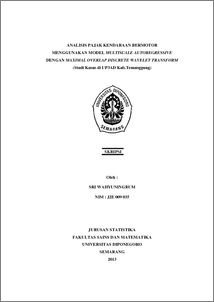ANALISIS PAJAK KENDARAAN BERMOTOR MENGGUNAKAN MODEL MULTISCALE AUTOREGRESSIVE DENGAN MAXIMAL OVERLAP DISCRETE WAVELET TRANSFORM (Studi Kasus di UP3AD Kab.Temanggung)

WAHYUNINGRUM, SRI (2013) ANALISIS PAJAK KENDARAAN BERMOTOR MENGGUNAKAN MODEL MULTISCALE AUTOREGRESSIVE DENGAN MAXIMAL OVERLAP DISCRETE WAVELET TRANSFORM (Studi Kasus di UP3AD Kab.Temanggung). Undergraduate thesis, UNIVERSITAS DIPONEGORO.Preview
PDF
184Kb

Abstract

Time series analysis is applied in many fields, one of them is in the economic field. In this paper will consider analysis of the time series on data income taxes motor vehicles UP3AD Kab.Temanggung using Maximal Overlap Wavelet Transform Discrete (MODWT). Data time series decomposed using wavelet transform, namely MODWT with filter Haar and D4. From this transformation wavelet coefficients and scales coefficients are used for the modeling of time series. Modeling is done using the Multiscale Autoregressive (MAR) forecasting to get period ahead. Results of analysis showed that the model MAR with filter D4 is better than on the model MAR with filter Haar. Keywords: Maximal Overlap Discrete Wavelet Transform (MODWT), Time Series, Multiscale Autoregressive (MAR)

Item Type: Thesis (Undergraduate) H Social Sciences > HA Statistics Faculty of Science and Mathematics > Department of Statistics 42329 Mr Hasbi Yasin 19 Feb 2014 14:18 04 Mar 2014 11:23

Repository Staff Only: item control page